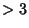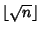## Eratosthenes SieveAn Algorithm for making tables of Primes. Sequentially write down the Integers from 2 to the highest numberyou wish to include in the table. Cross out all numberswhich are divisible by 2 (every second number). Find the smallest remaining number. It is 3. So cross out all numberswhich are divisible by 3 (every third number). Find the smallest remaining number. It is 5. So cross out all numberswhich are divisible by 5 (every fifth number).

Continue until you have crossed out all numbers divisible by, whereis the Floor Function. The numbers remaining are Prime. This procedure is illustrated in the above diagram which sieves up to 50, and therefore crosses out Primes up to. If the procedure is then continued up to, then the number of cross-outs gives the number of distinct Prime factors of each number.

References

Conway, J. H. and Guy, R. K. The Book of Numbers. New York: Springer-Verlag, pp. 127-130, 1996.

Ribenboim, P. The New Book of Prime Number Records. New York: Springer-Verlag, pp. 20-21, 1996.